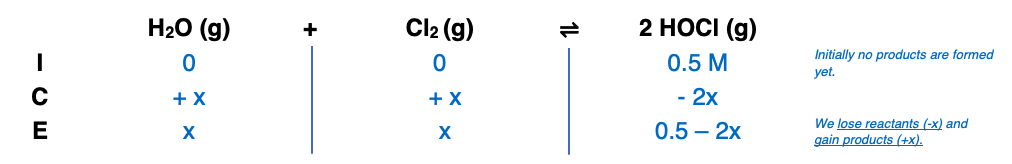# Problem: At 25ºC, K = 0.090 for the reactionH2O(g) + Cl2O(g) ⇌ 2HOCl(g)Calculate the concentrations of all species at equilibrium for each of the following cases.b. 1.0 mole of pure HOCl is placed in a 2.0-L flask.

🤓 Based on our data, we think this question is relevant for Professor Lazaridis' class at CCNY.

###### FREE Expert Solution

Step 1

= 0.5 M

Step 2Step 3###### Problem Details

At 25ºC, K = 0.090 for the reaction

H2O(g) + Cl2O(g) ⇌ 2HOCl(g)

Calculate the concentrations of all species at equilibrium for each of the following cases.

b. 1.0 mole of pure HOCl is placed in a 2.0-L flask.